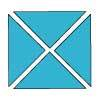#### You may also like### Chocolate

There are three tables in a room with blocks of chocolate on each. Where would be the best place for each child in the class to sit if they came in one at a time?### Four Triangles Puzzle

Cut four triangles from a square as shown in the picture. How many different shapes can you make by fitting the four triangles back together?### Cut it Out

Can you dissect an equilateral triangle into 6 smaller ones? What number of smaller equilateral triangles is it NOT possible to dissect a larger equilateral triangle into?

# Multiplication Square Jigsaw

##### Age 7 to 11Challenge Level

How are the numbers in the multiplication square made?
What is the smallest number in the square? Where does that go? Can you find the piece with it on?
How about the largest number? Where would that be placed in the grid?
What patterns do you notice in the pieces you have put in? Can you continue the patterns and therefore fit some more pieces in?